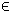## 12MILLS Problem

The mixed-integer linear least squares (mills) problem is defined as
 min x
f(x) =
1
2
|| C xd ||

s/t
 xL ≤ x ≤ xU, bL ≤ A x ≤ bU
(14)
where x, xL, xURn, dRM, CRM × n, ARm1 × n and bL,bURm1. The variables xI, the index subset of 1,...,n, are restricted to be integers.

The following file defines and solves a problem in TOMLAB.

File: tomlab/quickguide/millsQG.m

Open the file for viewing, and execute millsQG in Matlab.
```% millsQG is a small example problem for defining and solving
% mixed-integr linear least squares using the TOMLAB format.
Name='MILLS test example';           % Problem name, not required.
n = 10;
x_L = zeros(n,1);                    % Lower bounds on x
x_U = 20*ones(n,1);                  % Upper bounds on x

% Matrix defining linear constraints
A   = [ ones(1,n) ; 1:10; -2 1 -1 1 -1 1 -1 1 -1 1];
b_L = [35  -inf  29]';    % Lower bounds on the linear inequalities
b_U = [inf  120 210]';    % Upper bounds on the linear inequalities

% Vector m x 1 with observations in objective ||Cx -y(t)||
y = 2.5*ones(10,1);

% Matrix m x n in objective ||Cx -y(t)||
C = [ ones(1,n); 1 2 1 1 1 1 2 0 0 0; 1 1 3 1 1 1 -1 -1 -3 1; ...
1 1 1 4 1 1 1 1 1 0;1 1 1 3 1 1 1 1 1 0;1 1 2 1 1 0 0 0 -1 1; ...
1 1 1 1 0 1 1 1 1 0;1 1 1 0 1 1 1 1 1 1;1 1 0 1 1 1 2 2 3 0; ...
1 0 1 1 1 1 0 2 2 1];

% Starting point.
x_0 = 1./[1:n]';

% x_min and x_max are only needed if doing plots.
x_min = -ones(n,1);
x_max =  ones(n,1);